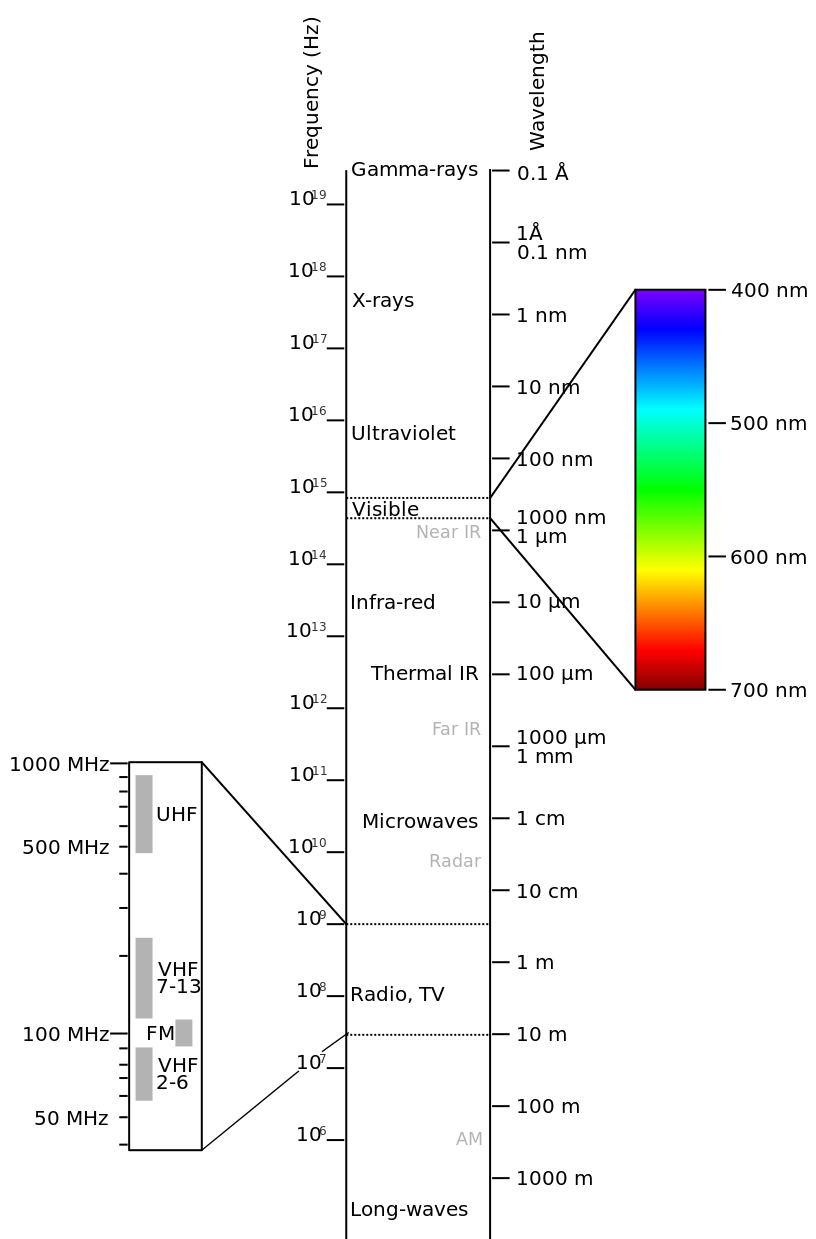1.2: Electromagnetic Spectrum

Electromagnetic fields exist at frequencies from DC (0 Hz) to at least 1020 Hz – that’s at least 20 orders of magnitude! At DC, electromagnetics consists of two distinct disciplines: electrostatics, concerned with electric fields; and magnetostatics, concerned with magnetic fields. At higher frequencies, electric and magnetic fields interact to form propagating waves. Waves having frequencies within certain ranges are given names based on how they manifest as physical phenomena. These names are (in order of increasing frequency): radio, infrared (IR), optical (also known as “light”), ultraviolet (UV), X-rays, and gamma rays (γ-rays). See Table $$\PageIndex{1}$$ and Figure $$\PageIndex{1}$$ for frequency ranges and associated wavelengths.

Definition: electromagnetic spectrum

The term electromagnetic spectrum refers to the various forms of electromagnetic phenomena that exist over the continuum of frequencies

The speed (properly known as “phase velocity”) at which electromagnetic fields propagate in free space is given the symbol $$c$$, and has the value ∼= 3.00 × 108 m/s. This value is often referred to as the “speed of light.” While it is certainly the speed of light in free space, it is also speed of any electromagnetic wave in free space. Given frequency $$f$$, wavelength is given by the expression

$\underbrace{\lambda = \frac { c } { f }}_{\text{in free space}}$

Table $$\PageIndex{1}$$ shows the free space wavelengths associated with each of the regions of the electromagnetic spectrum. This book presents a version of electromagnetic theory that is based on classical physics. This approach works well for most practical problems. However, at very high frequencies, wavelengths become small enough that quantum mechanical effects may be important. This is usually the case in the X-ray band and above. In some applications, these effects become important at frequencies as low as the optical, IR, or radio bands. (A prime example is the photoelectric effect; see “Additional References” below.) Thus, caution is required when applying the classical version of electromagnetic theory presented here, especially at these higher frequencies.

Table $$\PageIndex{1}$$: The electromagnetic spectrum. Note that the indicated ranges are arbitrary but consistent with common usage.
Regime Frequency Range Wavelength Range
γ-Ray $$\mathrm{> 3 \times 10^{19} \; Hz}$$ < 0.01 nm
X-Ray $$\mathrm{3 \times 10^{16} \; Hz \; – \; 3 \times 10^{19} \; Hz}$$ 10–0.01 nm
Ultraviolet (UV) $$\mathrm{2.5 \times 10^{15} \; – \; 3 \times 10^{16} \; Hz}$$ 120–10 nm
Optical $$\mathrm{4.3 \times 10^{14} \; – \; 2.5 \times 10^{15} \; Hz}$$ 700–120 nm
Infrared (IR) $$\mathrm{300 \; GHz \; – \; 4.3 \times 10^{14} \; Hz}$$ 1 mm – 700 nm
Radio $$\mathrm{3 \; kHz – 300 \; GHz}$$ 100 km – 1 mm

The radio portion of the electromagnetic spectrum alone spans 12 orders of magnitude in frequency (and wavelength), and so, not surprisingly, exhibits a broad range of phenomena. This is shown in Figure $$\PageIndex{1}$$.Figure $$\PageIndex{1}$$: Electromagnetic Spectrum.

For this reason, the radio spectrum is further subdivided into bands as shown in Table $$\PageIndex{2}$$. Also shown in Table $$\PageIndex{2}$$ are commonly-used band identification acronyms and some typical applications. Similarly, the optical band is partitioned into the familiar “rainbow” of red through violet, as shown in Figure $$\PageIndex{1}$$ and Table $$\PageIndex{3}$$. Other portions of the spectrum are sometimes similarly subdivided in certain applications.

Table $$\PageIndex{2}$$: The radio portion of the electromagnetic spectrum, according to a common scheme for naming ranges of radio frequencies. WLAN: Wireless local area network, LMR: Land mobile radio, RFID: Radio frequency identification
Band Frequencies Wavelengths Typical Applications
EHF 30-300 GHz 10–1 mm 60 GHz WLAN, Point-to-point data links
SHF 3–30 GHz 10–1 cm Terrestrial & Satellite data links, Radar
UHF 300–3000 MHz 1–0.1 m TV broadcasting, Cellular, WLAN
VHF 30–300 MHz 10–1 m FM & TV broadcasting, LMR
HF 3–30 MHz 100–10 m Global terrestrial comm., CB Radio
MF 300–3000 kHz 1000–100 m AM broadcasting
LF 30–300 kHz 10–1 km Navigation, RFID
VLF 3–30 kHz 100–10 km Navigation

Table $$\PageIndex{3}$$: The optical portion of the electromagnetic spectrum.
Band Frequencies Wavelengths
Violet 668–789 THz 450–380 nm
Blue 606–668 THz 495–450 nm
Green 526–606 THz 570–495 nm
Yellow 508–526 THz 590–570 nm
Orange 484–508 THz 620–590 nm
Red 400–484 THz 750–620 nm